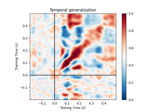# mne.decoding.Scaler¶

class mne.decoding.Scaler(info=None, scalings=None, with_mean=True, with_std=True)[source]

Standardize channel data.

This class scales data for each channel. It differs from scikit-learn classes (e.g., `sklearn.preprocessing.StandardScaler`) in that it scales each channel by estimating μ and σ using data from all time points and epochs, as opposed to standardizing each feature (i.e., each time point for each channel) by estimating using μ and σ using data from all epochs.

Parameters
infoinstance of `Info` | `None`

The measurement info. Only necessary if `scalings` is a dict or None.

scalings

Scaling method to be applied to data channel wise.

with_meanbool, default `True`

If True, center the data using mean (or median) before scaling. Ignored for channel-type scaling.

with_stdbool, default `True`

If True, scale the data to unit variance (`scalings='mean'`), quantile range (`scalings='median`), or using channel type if `scalings` is a dict or None).

Methods

 `__hash__`(/) Return hash(self). `fit`(epochs_data[, y]) Standardize data across channels. `fit_transform`(epochs_data[, y]) Fit to data, then transform it. `get_params`([deep]) Get parameters for this estimator. `inverse_transform`(epochs_data) Invert standardization of data across channels. `set_params`(**params) Set the parameters of this estimator. `transform`(epochs_data) Standardize data across channels.
fit(epochs_data, y=None)[source]

Standardize data across channels.

Parameters
epochs_data`array`, shape (n_epochs, n_channels, n_times)

The data to concatenate channels.

y`array`, shape (n_epochs,)

The label for each epoch.

Returns
selfinstance of `Scaler`

The modified instance.

Examples using `fit`:fit_transform(epochs_data, y=None)[source]

Fit to data, then transform it.

Fits transformer to epochs_data and y and returns a transformed version of epochs_data.

Parameters
epochs_data`array`, shape (n_epochs, n_channels, n_times)

The data.

y`None` | `array`, shape (n_epochs,)

The label for each epoch. Defaults to None.

Returns
X`array`, shape (n_epochs, n_channels, n_times)

The data concatenated over channels.

Notes

This function makes a copy of the data before the operations and the memory usage may be large with big data.

Examples using `fit_transform`:get_params(deep=True)[source]

Get parameters for this estimator.

Parameters
deepbool, optional

If True, will return the parameters for this estimator and contained subobjects that are estimators.

Returns
params`dict`

Parameter names mapped to their values.

inverse_transform(epochs_data)[source]

Invert standardization of data across channels.

Parameters
epochs_data`array`, shape (n_epochs, n_channels, n_times)

The data.

Returns
X`array`, shape (n_epochs, n_channels, n_times)

The data concatenated over channels.

Notes

This function makes a copy of the data before the operations and the memory usage may be large with big data.

Examples using `inverse_transform`:set_params(**params)[source]

Set the parameters of this estimator.

The method works on simple estimators as well as on nested objects (such as pipelines). The latter have parameters of the form `<component>__<parameter>` so that it’s possible to update each component of a nested object.

Parameters
**params`dict`

Parameters.

Returns
instinstance

The object.

transform(epochs_data)[source]

Standardize data across channels.

Parameters
epochs_data`array`, shape (n_epochs, n_channels[, n_times])

The data.

Returns
X`array`, shape (n_epochs, n_channels, n_times)

The data concatenated over channels.

Notes

This function makes a copy of the data before the operations and the memory usage may be large with big data.

Examples using `transform`:## Examples using `mne.decoding.Scaler`¶